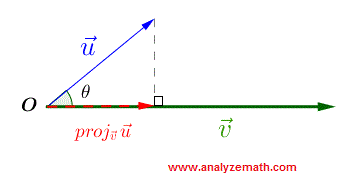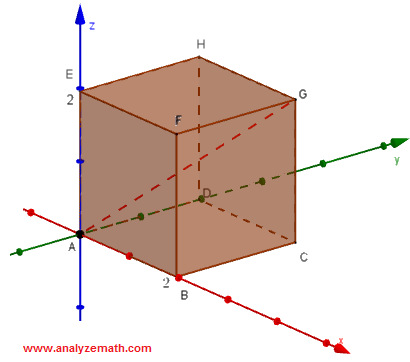# Scalar and Cross Products of 3D Vectors

The scalar (or dot product) and cross product of 3 D vectors are defined and their properties discussed and used to solve 3D problems.

## Scalar (or dot) Product of Two Vectors

The scalar (or dot) product of two vectors $\vec{u}$ and $\vec{v}$ is a scalar quantity defined by:

$\vec{u} \cdot \vec{v} = || \vec{u} || \, || \vec{v} || \cos \theta$

where $|| \vec{u} ||$ is the magnitude of vector $\vec{u}$, $|| \vec{v} ||$ is the magnitude of vector $\vec{v}$ and $\theta$ is the angle between the vectors $\vec{u}$ and $\vec{v}$.
If the components of vectors $\vec{u}$ and $\vec{v}$ are known: $\vec{u} = (u_x , u_y ,u_z)$ and $\vec{v} = (v_x , v_y , v_z)$, it can be shown that the scalar product may be expressed as follows:
$\vec{u} \cdot \vec{v} = u_x v_x + u_y v_y + u_zv_z$

## Theorems on Scalar Products

If $\vec{u}$, $\vec{v}$ and $\vec{w}$ are vectors and k is a scalar, then
1) $\vec{u} \cdot \vec{v} = \vec{v} \cdot \vec{u}$
2) $\vec{u} \cdot (\ \vec{v} + \vec{w} ) = \vec{u} \cdot \vec{v} + \vec{u} \cdot \vec{w}$
3) $\vec{u} \cdot \vec{u} = ||\vec{u} ||^2$
4) $\vec{u} \cdot \vec{v} = 0 \;$ if and only if $\; \theta = \pi/2$, if both $\vec{u}$ and $\vec{v}$ are non zero vectors.
5) $(k \vec{u}) \cdot \vec{v} = \vec{u} \cdot ( k\vec{v}) = k ( \vec{u} \cdot \vec{v} )$
6) $|\vec{u} \cdot \vec{v} | \le ||\vec{u}|| ||\vec{v}||$
7) $||\vec{u} + \vec{v} || \le ||\vec{u}|| + ||\vec{v}||$

### Example 1

Approximate, to the nearest degree, the angle between the vectors $\vec{v}=<-2,3,1> \text{and} \vec{u}= <0,-1,4>$.

### solution

Express the scalar product of the two vectors using the magnitude and angle $\theta$ between them and the coordinates as follows:
$\vec{v} \cdot \vec{u} = ||\vec{v} || || \vec{u} || cos \theta = (-2)(0) + (3)(-1) + (1)(4) = 1$
$||\vec{v} || = \sqrt{(-2)^2+3^2+1^2} = \sqrt{14}$
$||\vec{u} || = \sqrt{0^2+(-1)^2+4^2} = \sqrt{17}$
$cos\theta = \dfrac{1}{||\vec{v} || || \vec{u} ||} = \dfrac{1}{\sqrt{14}\sqrt{17}}$
$\theta = \arccos(\dfrac{1}{\sqrt{14}\sqrt{17}} ) \approx 86^{\circ}$

More explanations on finding the angle between vectors on a video.

### Example 2

Find $a$ so that the vectors $\lt a,-6,3 \gt$ and $<1,0,-2>$ are perpendicular.

### solution

For two vectors to be perpendicular, their scalar product must be equal to zero.
$\lt a,-6,3 \gt \cdot <1,0,-2> = a(1) + (-6)(0)+(3)(-2) = a - 6 = 0$
Solve for a
$a = 6$

## Scalar and Vector Projection of a Vector onto Another

In many applications, it is important to find the component of a vector in the direction of another vector. As shown below, vector $\vec{u}$ is projected onto vector $\vec{v}$ by dropping a perpendicular from the terminal point of $\vec{u}$ to the line through $\vec{v}$. The component of $\vec{u}$ along $\vec{v}$ is a scalar quantity called the scalar projection and is given by

$\text{comp}_{\vec{v}}\vec{u} = ||\vec{u}|| \cos \theta$
.
The vector projection of $\vec{u}$ on $\vec{v}$ is a
vector quantity obtained by multiplying the component $\text{comp}_{\vec{v}}\vec{u}$ by the unit vector in the direction of vector $\vec{v}$ and is given by
$\text{proj}_{\vec{v}}\vec{u} = ||\vec{u}|| \cos \theta \dfrac{\vec{v}}{||\vec{v}||} = \dfrac{\vec{u}\cdot\vec{v}}{||v||^2} \vec{v}$
.## Cross (or vector) Product of Two Vectors

The cross (or vector) product of two vectors $\vec{u} = (u_x , u_y ,u_z)$ and $\vec{v} = (v_x , v_y , v_z)$ is a vector quantity defined by:

$\vec{u} \times \vec{v} = {\begin{vmatrix}\vec{i}& \vec{j} &\vec{k} \\ u_x & u_y & u_z \\ v_x & v_y & v_z \end{vmatrix}} = {\begin{vmatrix} u_y & u_z \\ v_y & v_z \end{vmatrix}} \vec{i} - {\begin{vmatrix}u_x & u_z\\ v_x & v_z\end{vmatrix}} \vec{j} + {\begin{vmatrix}u_x & u_y\\ v_x & v_y\end{vmatrix}} \vec{k}$

The cross product $\vec{u} \times \vec{v}$ is perpendicular to both $\vec{v}$ and $\vec{u}$The right hand rule, to find the direction of the cross product, is as follows: point the index in the direction of $\vec {u}$, the middle finger in the direction of $\vec{v}$ and the direction of the cross product $\vec {u} \times \vec {v}$ is in the same direction as that of the thumb.## Theorems on Cross Products

If $\vec{u}$, $\vec{v}$ and $\vec{w}$ are vectors and k is a scalar, then
1) The cross product $\vec{u} \times \vec{v}$ is perpendicular to both $\vec{v}$ and $\vec{u}$
2) $\vec{u} \times \vec{v} = - \vec{v} \times \vec{u}$
3) $\vec{u} \times \vec{v} = 0$ if and only if $\vec{u}$ and $\vec{v}$ are parallel , if both $\vec{u}$ and $\vec{v}$ are non zero vectors.
4) $\vec{u} \times (\ \vec{v} + \vec{w} ) = \vec{v} \times \vec{u} + \vec{u} \times \vec{w}$
5) $(k \vec{u}) \times \vec{v} = \vec{u} \times ( k\vec{v}) = k ( \vec{u} \times \vec{v} )$
6) $||\vec{u} \times \vec{v} || = ||\vec{u}|| ||\vec{v}|| sin \theta$ , where $\theta$ is the angle between $\vec{u}$ and $\vec{v}$.

## Area of a Parallelogram

A parallelogram is a quadrilateral (4 sides) with opposite sides parallel. In the figure below is shown the parallelogram A, B, C and D. Hence, we have equality between the vectors.
$\vec{AB} = \vec{DC}$ and $\vec{AD} = \vec{BC}$
The area of the parallelagram is given by $|| \vec{AB} \times \vec{AD} ||$The area of a triangle may be calculated as half the area the corresponding parallelogram.

## Volume of a Parallelepiped

A parallelepiped is a 3d figure formed by 6 parallelograms as shown in the figure below. We have equality between several vectors.
$\vec{AE} = \vec{DH} = \vec{CG} = \vec{BF} = \vec{u}$
$\vec{AD} = \vec{BC} = \vec{EH} = \vec{FG} = \vec{v}$
$\vec{AB} = \vec{DC} = \vec{EF} = \vec{HG} = \vec{w}$
The volume V of the parallelepiped is given by
V $= |\vec{u}\cdot (\vec{v} \times \vec{w})| = | \vec{v}\cdot (\vec{w} \times \vec{u})| = | \vec{w}\cdot (\vec{v} \times \vec{u})|$### Example 3

Calculate the cross product of the vectors $\vec{u} = <1,1,3>$ and $\vec{v} = <1,0,2>$.

A video on how to find the Cross Product of Two Vectors with detailed explanations.

### solution

$\vec{u} \times \vec{v} = {\begin{vmatrix}\vec{i}& \vec{j} &\vec{k} \\ 1 & 1 & 3 \\ 1 & 0 & 2 \end{vmatrix}} = {\begin{vmatrix} 1 & 3 \\ 0 & 2 \end{vmatrix}} \vec{i} - {\begin{vmatrix}1 & 3\\ 1 & 2\end{vmatrix}} \vec{j} + {\begin{vmatrix}1 & 1\\ 1& 0\end{vmatrix}} \vec{k} = 2\vec{i} + \vec{j} -\vec{k}$

### Example 4

Find two unit vectors perpendicular to the vectors $\vec{u} = \lt 1,-2,1 \gt$ and $\vec{v} = <-2,0,4>$.

### solution

The cross product $\vec{w} = \vec{u} \times \vec{v}$ is a vector perpendicular to both vectors $\vec{u} \; \text{and} \; \vec{v}$.
Let us calculte $\vec{u} \times \vec{v}$ as follows:
$\vec{w} = \vec{u} \times \vec{v} = {\begin{vmatrix}\vec{i}& \vec{j} &\vec{k} \\ 1 & -2 & 1 \\ -2 & 0 & 4 \end{vmatrix}} = {\begin{vmatrix} -2 & 1 \\ 0 & 4 \end{vmatrix}} \vec{i} - {\begin{vmatrix}1 & 1\\ -2 & 4\end{vmatrix}} \vec{j} + {\begin{vmatrix}1 & -2\\ -2 & 0\end{vmatrix}} \vec{k} = -8\vec{i} - 6 \vec{j} - 4 \vec{k}$

We now need to find a unit vector $\vec{u_1}$ in the same direction as $\vec{w}$ and is given by
$\vec{u_1} = \dfrac{1}{||\vec{w}||} \vec{w}$
and a second unit vector $\vec{u_2}$ in the opposite direction of $\vec{w}$ and is given by
$\vec{u_2} = -\vec{u_1}$
$||\vec{w}|| = \sqrt{(-8)^2+(- 6)^2+(- 4)^2 } = 2\sqrt{29}$
$\vec{u_1} = \dfrac{1}{2\sqrt{29}} (-8\vec{i} - 6 \vec{j} - 4 \vec{k}) = -\dfrac{4}{\sqrt{29}}\vec{i} -\dfrac{3}{\sqrt{29}}\vec{j}-\dfrac{2}{\sqrt{29}}\vec{k}$
$\vec{u_2} = \dfrac{4}{\sqrt{29}}\vec{i} + \dfrac{3}{\sqrt{29}}\vec{j}+ \dfrac{2}{\sqrt{29}}\vec{k}$

### Example 5

Explain why the following statement are not true.
a) $\vec{u} \times \vec{u} = ||\vec{u}||^2$
b) $\vec{u} \cdot (\vec{u} \times \vec{w} )= (\vec{u} \cdot \vec{u}) \times \vec{w}$

### solution

a) The left side $\vec{u} \times \vec{u}$ is a cross product and the result is a vector. The right side $||\vec{u}||^2$ is a scalar quantity. A vector and a scalar cannot be compared.
b) The left side $\vec{u} \cdot (\vec{u} \times \vec{w} )$ is a scalar product of $\vec{u}$ and $(\vec{u} \times \vec{w} )$ and the result is a scalar. The right side is the product of a scalar quantity $\vec{u} \cdot \vec{u}$ and vector $\vec{w}$ and the result is a vector. A scalar and a vector cannot be compared.

Detailed Solutions and explanations to these questions.
1) Calculate $\vec{u} \cdot (\vec{u} \times \vec{v})$ given that $\vec{u} = \lt a,b,c \gt$ and $\vec{v} = \lt d,e,f \gt$.
2) Find $k$ so that vectors $\vec{u} = \lt -2,-k,1 \gt$ and $\vec{v} = <8,-2,-3>$ are perpendicular
3)Find $k$ so that the vectors $\vec{u} = \lt -3,2,-2 \gt$, $\vec{v} = <2,1,k>$ and $\vec{w} = <-1,3,-5>$ are on the same plane (or coplanar)?
4) Find angle $\theta$ between the vectors $\vec{u} = \lt 2,0,1 \gt$ and $\vec{v} = <8,-2,-3>$.
5) Find the vector projection of $\vec{u} = \lt -1,-1,1 \gt$ onto $\vec{v} = <2,1,1>$.
6) Find that $k$ so that the points $A(-1,2,k)$, $B(-3,6,3)$ and $C(1,3,6)$ are the vertices of a right triangle with a right angle at $A$.
7) Given vector $\vec{v} = \lt 3,-1,-2 \gt$, find the vector $\vec{u}$ such that $\vec{v} \times \vec{u} = <4,2,5>$ and $||\vec{u}|| = 3$)
8) Points A, B, C and D forms a parallelogram.
a)Find the coordinates of point D.
b)Find the area of a parallelogram.9) In the cube below find the angle between the diagonals AG and BH.10) Find a vector that is orthogonal to the plane containing the points A(1,2,-3), B(0,-2,1) and C(-2,0,1).
11) Find the area of the triangle whose vertices are the points A(1,0,-3), B(1,-2,0) and C(0,2,1).
12)Find the volume of the parallelepiped shown below.Detailed
Solutions and explanations to these questions.